Courses
Courses for Kids
Free study material
Free LIVE classes
More

# List of Math Words That Start With Y - Self Development Journey for KidsThere are many words in Mathematics that begin with the letter Y. It is easier to remember these together and distinctly so that kids do not forget or mix up the meanings of these words, which can be the case if these are not revised or kept in mind separately. So let us study the meanings and uses of some words in Mathematics starting with the letter Y.

## List of Maths Words Starting with the Letter Y

Below is a list of some Math words that start with the letter Y.

Y-Axis: There are two axes in a coordinate system, the x-axis and the y-axis. The vertical axis is also known as the y-axis.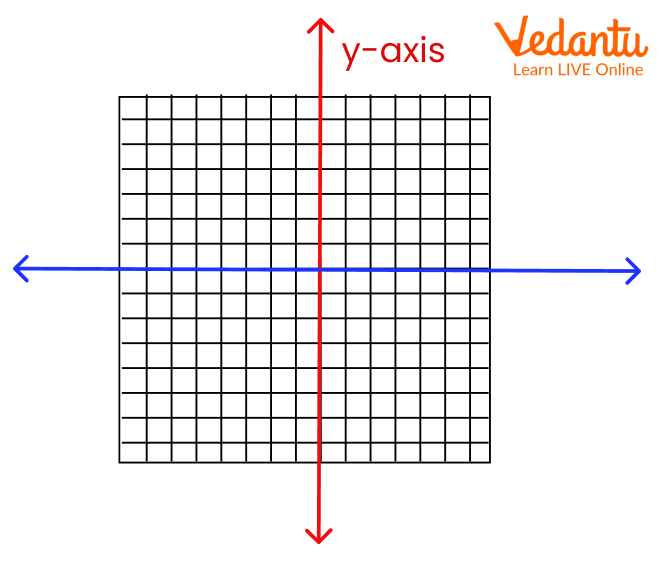Y-Axis

Y-Coordinate: The coordinates tell us how far we need to travel in a direction from the origin to reach a given point. The y-coordinate gives us the measure of how far we need to move vertically from the origin to reach a location. The y-coordinate can be positive or negative. The positive y-coordinate will tell us to go north and the negative y-coordinate will tell us to move south from a point. We generally denote the y-coordinate as the second number after the comma. For instance, in a point (1,2) in a 2-dimensional coordinate plane, 2 is the y-coordinate.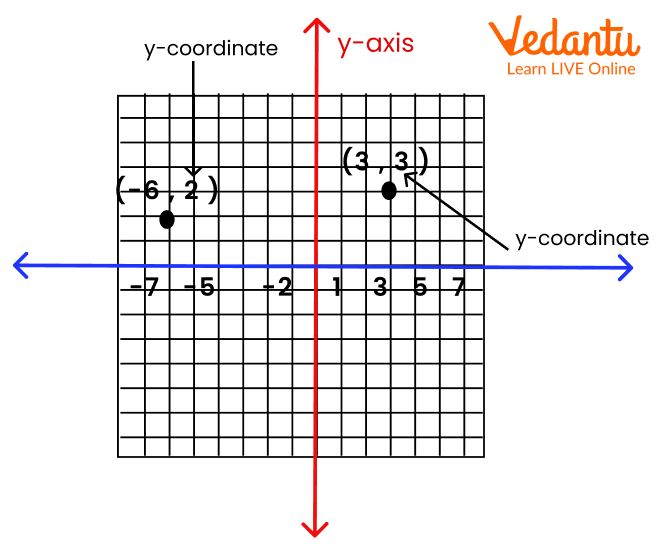Y-Coordinate

Yard: A yard in Maths is different from a yard as in a field or garden. It is a unit of linear measurement in the coordinate system that is used to measure lengths and distances. 1 yard is equal to 3 feet or 36 inches. Yards are used to measure fields (farms or even football fields). They are also used to measure small distances between places.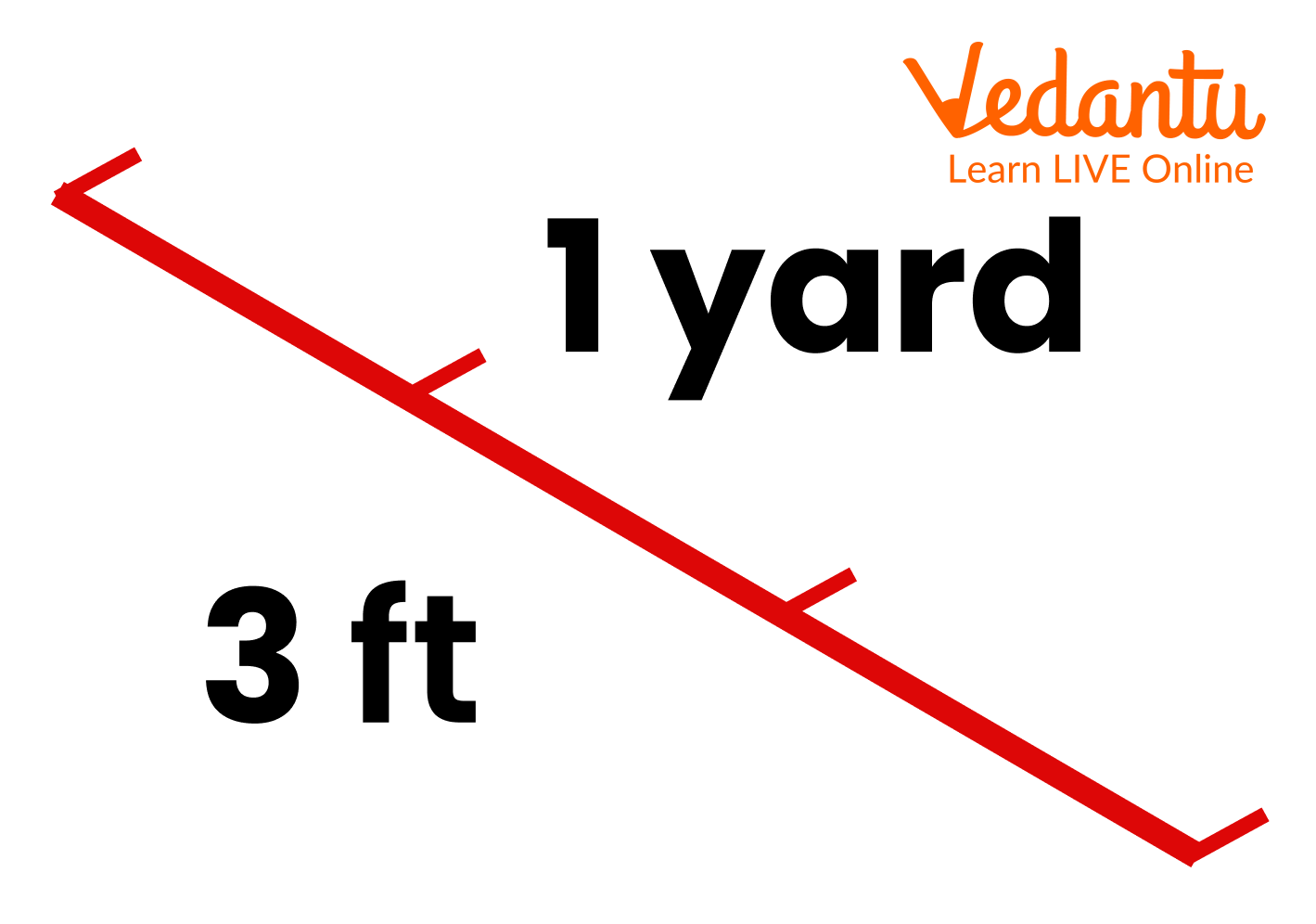3 Feet Equals 1 Yard

Yardstick: A yardstick is basically a measuring stick that is graduated at equal lengths and measures a total of up to a yard. It can be imagined as a scale that we use to measure small lengths.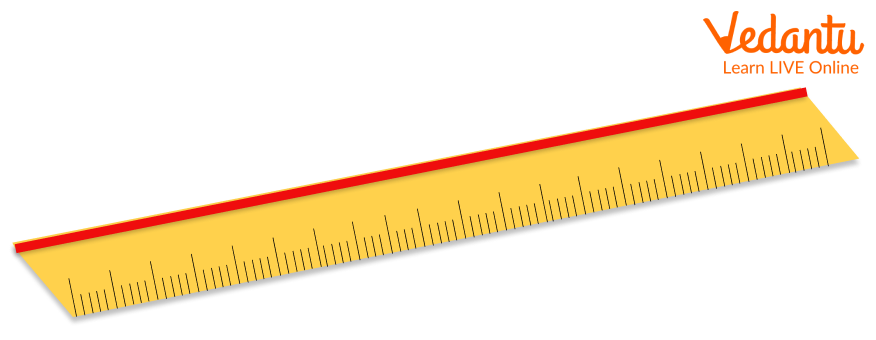A Yardstick

Year: A year is a period of 365 days normally and 366 days in a leap year (which comes every 4 years). A year has 12 months or 52 weeks. A year can also be considered as the time taken by the Earth to complete one full revolution around the Sun. Your birthday comes every year, so we measure age in years as well.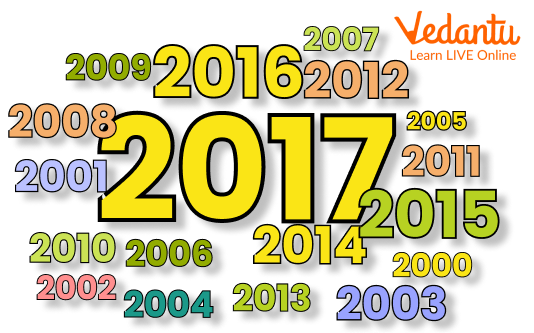Years

## Conclusion

The words discussed in this article are very important to remember in Maths and they all start with the letter Y. So, it will be actually really easy to memorise them together. However, it can be easy to confuse some of them. So, they should be studied separately as well.

Last updated date: 27th Sep 2023
Total views: 137.7k
Views today: 3.37k

## FAQs on List of Math Words That Start With Y - Self Development Journey for Kids

1. How is the y-axis different from a y-coordinate?

It is easy to confuse between the y-axis and the y-coordinate. The difference is that the y-axis is the vertical axis in a 2-dimensional coordinate system while the y-coordinate is an indication of how far we need to move in the vertical direction from a point.